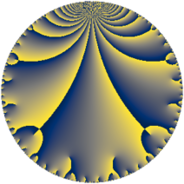# Properties

 Label 441.6.hLevel $441$ Weight $6$ Character orbit 441.h Rep. character $\chi_{441}(214,\cdot)$ Character field $\Q(\zeta_{3})$ Dimension $392$ Sturm bound $336$

# Related objects

## Defining parameters

 Level: $$N$$ $$=$$ $$441 = 3^{2} \cdot 7^{2}$$ Weight: $$k$$ $$=$$ $$6$$ Character orbit: $$[\chi]$$ $$=$$ 441.h (of order $$3$$ and degree $$2$$) Character conductor: $$\operatorname{cond}(\chi)$$ $$=$$ $$63$$ Character field: $$\Q(\zeta_{3})$$ Sturm bound: $$336$$

## Dimensions

The following table gives the dimensions of various subspaces of $$M_{6}(441, [\chi])$$.

Total New Old
Modular forms 576 408 168
Cusp forms 544 392 152
Eisenstein series 32 16 16

## Trace form

 $$392 q + 2 q^{2} + q^{3} + 6146 q^{4} - 101 q^{5} + 116 q^{6} + 48 q^{8} + 161 q^{9} + O(q^{10})$$ $$392 q + 2 q^{2} + q^{3} + 6146 q^{4} - 101 q^{5} + 116 q^{6} + 48 q^{8} + 161 q^{9} + 66 q^{10} - 381 q^{11} + 1550 q^{12} - 179 q^{13} + 2854 q^{15} + 94274 q^{16} - 2043 q^{17} - 980 q^{18} + 64 q^{19} - 2782 q^{20} + 60 q^{22} - 5240 q^{23} + 10746 q^{24} - 112501 q^{25} - 4798 q^{26} + 5551 q^{27} + 3126 q^{29} - 13257 q^{30} + 2170 q^{31} - 4282 q^{32} + 6979 q^{33} - 30 q^{34} - 2992 q^{36} + 2581 q^{37} - 22255 q^{38} - 6601 q^{39} - 1014 q^{40} - 46630 q^{41} - 9248 q^{43} - 37565 q^{44} - 59897 q^{45} - 9606 q^{46} + 69510 q^{47} + 111629 q^{48} + 146415 q^{50} - 47438 q^{51} - 9473 q^{52} - 26012 q^{53} + 111779 q^{54} - 22314 q^{55} - 99717 q^{57} + 5079 q^{58} + 186780 q^{59} + 251039 q^{60} - 96284 q^{61} + 301716 q^{62} + 1378352 q^{64} + 7368 q^{65} + 84614 q^{66} - 1244 q^{67} - 138522 q^{68} - 253152 q^{69} + 942 q^{71} - 297024 q^{72} + 42016 q^{73} - 32401 q^{74} - 122134 q^{75} + 3010 q^{76} + 383085 q^{78} - 97148 q^{79} - 190471 q^{80} - 298927 q^{81} - 30 q^{82} - 177090 q^{83} + 14571 q^{85} + 12277 q^{86} - 235181 q^{87} - 30849 q^{88} - 176927 q^{89} + 238307 q^{90} + 191714 q^{92} + 148888 q^{93} + 192738 q^{94} + 478114 q^{95} - 971 q^{96} + 34681 q^{97} + 403910 q^{99} + O(q^{100})$$

## Decomposition of $$S_{6}^{\mathrm{new}}(441, [\chi])$$ into newform subspaces

The newforms in this space have not yet been added to the LMFDB.

## Decomposition of $$S_{6}^{\mathrm{old}}(441, [\chi])$$ into lower level spaces

$$S_{6}^{\mathrm{old}}(441, [\chi]) \cong$$ $$S_{6}^{\mathrm{new}}(63, [\chi])$$$$^{\oplus 2}$$